## Least Square Estimation Method:

As we are going to seen that, the problem of power system state estimation is a special case of the more general problem of estimation of a random vector x from the numerical values of another related random vector y with relatively little statistical information being available for both x and y. In such cases, the method of Least Square Estimation may be utilized with good results and has accordingly been widely employed.

Assume that x is a vector of n random variables x1, x2, …, xn, that y is another vector of m (> n) random variables y1, y2, …, ym and both are related as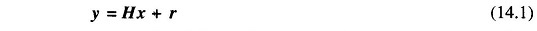where H is a known matrix of dimension m x n and r is a zero mean random variable of the same dimension as y. The vector x represents the variables to be estimated, while the vector y represents the variables whose numerical values are available. Equation (14.1) suggests that the measurement vector y is linearly related to the unknown vector x and in addition is corrupted by the vector r (error vector).

The problem is basically to obtain the best possible value of the vector x from the given values of the vector y. Since the variable r is assumed to be zero mean, one may take the expectation of Eq. (14.1) and get the relation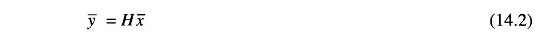where

• x̅, y̅ = expected value of x and y, respectively.

This shows that the load flow methods already could be used to estimate the mean values of the bus voltages. However, one would like to estimate the actual values of bus voltages rather than their averages.

One possible way of obtaining the best possible estimate of the vector x from y lies in the use of the method of Least Square Estimation (LSE). To develop this method, assume that x̂ represents the desired estimate of x so that ŷ given by the equationrepresents the estimate of the vector y. The error ŷ of the Least Square Estimation of y is then given by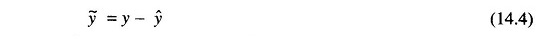The estimate x̂ is defined to be the LSE if it is computed by minimizing the Least Square Estimation index J given by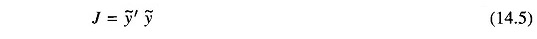From Eqs. (14.31) and (14.4), one gets the following expression for the index: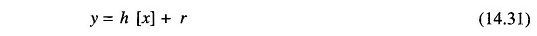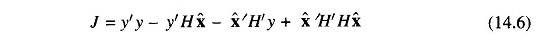For minimizing J = f(x̂), we must satisfy the following condition.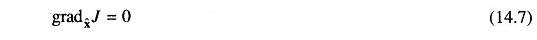It is easy to check that Eq. (14.7) leads to the following result.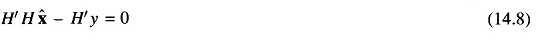This equation is called the ‘normal equation‘ and may be solved explicitly for the LSE of the vector x̂ as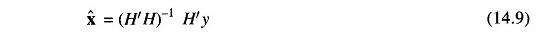Scroll to Top